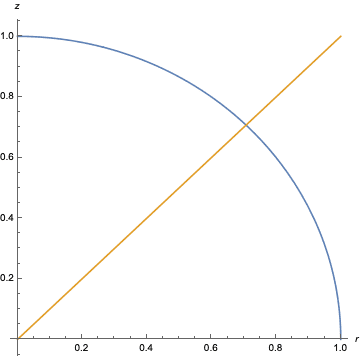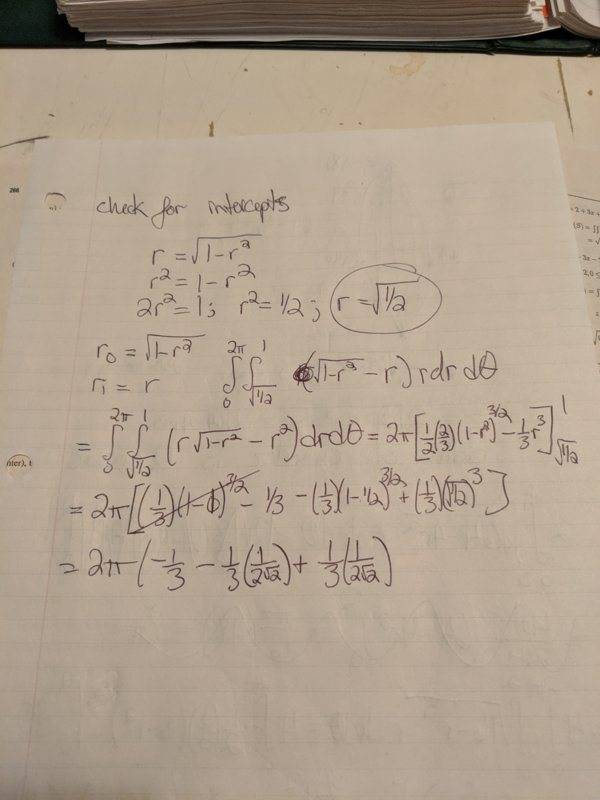# Help please in understanding the limits of this integration

snatchingthepi
Homework Statement:
Finding the volume above the cone z = sqrt(x^2 = y^2) and below sphere x^2 + y^2 + z^2 = 1
Relevant Equations:
Cone: z = sqrt(x^2 = y^2)

Sphere: x^2 + y^2 + z^2 = 1
So I can push this integral all the way to the end and see I get a negative volume.

I solve for the intercepts of the cone and sphere at r^2 = 1/2. Seeing this cone is inside the sphere and the sphere is around it, I figure I should integrate from sqrt(1/2) to 1 since we're dealing with a unit sphere, and these limits would give the area *above* the cone and *below* the sphere.

But when I check the solution manual I see the problem involves using radial limits from 0 to sqrt(1/2) but the equation that is integrated is exactly the same as mine. Using limits from 0 to root(1/2) would seem to me to give the area above the plane projection of the sphere and below the cone, not above the cone and below the sphere. Can someone help me understand where this is coming from?

Mentor
Problem Statement: Finding the volume above the cone z = sqrt(x^2 = y^2) and below sphere x^2 + y^2 + z^2 = 1
Relevant Equations: Cone: z = sqrt(x^2 = y^2)

Sphere: x^2 + y^2 + z^2 = 1

So I can push this integral all the way to the end and see I get a negative volume.

I solve for the intercepts of the cone and sphere at r^2 = 1/2. Seeing this cone is inside the sphere and the sphere is around it, I figure I should integrate from sqrt(1/2) to 1 since we're dealing with a unit sphere, and these limits would give the area *above* the cone and *below* the sphere.

But when I check the solution manual I see the problem involves using radial limits from 0 to sqrt(1/2) but the equation that is integrated is exactly the same as mine. Using limits from 0 to root(1/2) would seem to me to give the area above the plane projection of the sphere and below the cone, not above the cone and below the sphere. Can someone help me understand where this is coming from?
Can you show us the integral you're evaluating? Your description omits some details that would be helpful.

Staff Emeritus
Homework Helper
Does this plot help?snatchingthepiHere is my image of the integral I've computed. Barring an extra parentheses I've clearly dropped by accident at the end, I am trying to run it from sqrt(1/2) to 1. Looking at the graph above provided by vela (thank you) I see where everything intercepts, but I do not understand why the given solutions integrates from 0 to sqrt(1/2).

To get the area above the cone and below the sphere shouldn't I have to use limits of integration from sqrt(1/2) to 1?

Staff Emeritus